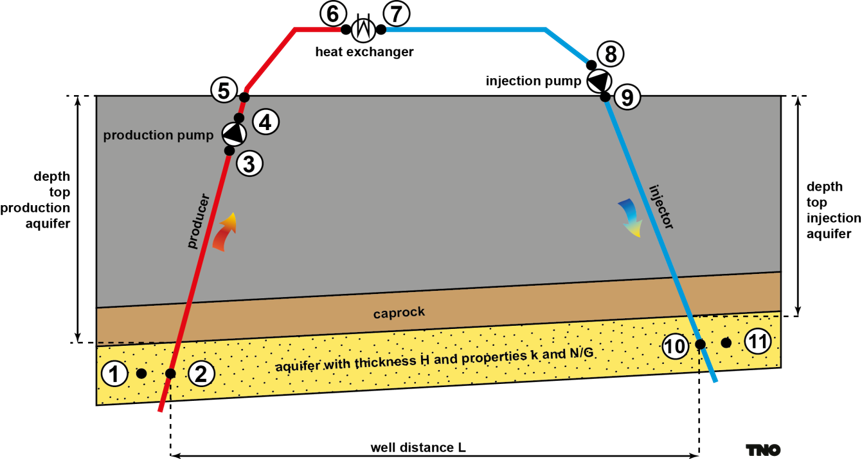# Technical model

The technical input for the sequential DoubletCalc1D calculation consists of a combination of maps, constant values and optimised values (see table). The depth and thickness maps are described in the Aquifers section, and the net-to-gross and permeability maps are described in the Aquifer properties section. The temperature is extracted from the 3D temperature model. The salinity of the brine is depth-dependent and calculated as 70,000/1,500 x depth [ppm].

The distance between two wells at aquifer level is optimized per aquifer and per XY location (grid cell). A large distance increases the resistance the water experiences when moving to a well. A small distance causes early thermal breakthrough in the production well. The maximum allowed cooling of the production water after 50 years is set to 10% of the difference between the initial aquifer temperature and the return temperature. The well distance is minimized, while at the same time the maximum cooling is not exceeded.Figure 8. Schematic overview of a geothermal doublet used in DoubletCalc1D

Next, the pump pressure is optimized. A higher pump pressure results in a higher flow rate and therefore a higher geothermal power. On the other hand, a higher pump pressure increases the costs of operating the pump and possibly results in a higher cost per unit energy. Furthermore, the pump pressure is limited by the pump specifications (set to 300 bar for ThermoGIS v2.0), and the State Supervision of the Mines protocol ‘Definition maximum injection pressure for geothermal production’ (in Dutch). Within these constraints, the pump pressure is optimized towards a minimum cost per unit energy.

The "well trajectory curvature factor" is used to calculate the along-hole well depth of both wells from the vertical depth and the well distance. The internal casing diameter between the reservoir and the surface level is 8.5". The reservoir section also has an 8.5" diameter, and is assumed to be open hole. The well penetration angle in the reservoir is assumed to be 45° (deviation from vertical). In order to simulate this deviation in the flow calculation, a negative skin is assumed, which has a value of -1 for a 45° angle.

The most important results of the technical analysis are maps for each aquifer and stack depicting the geothermal power [MWthermisch], the flow rate [m³/hr] and the production temperature [°C].